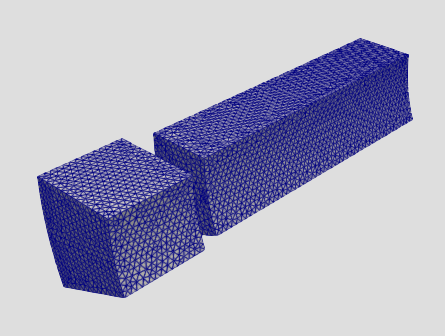# Extrapolate the Data to get Full Grid

Hi,

I want to extrapolate my partial data to get full grid.

Here is the full Grid:

I want to get the full grid from the grid below;It is just 1/16th of the full grid. I need to copy and translate the partial grid by 16 times. But I couldn’t find any filter that might be useful for my problem?

I don’t know of any way to do this automatically, but you can use the Transform filter to create a rotated copy of the data. Assuming that the X axis is the center of rotation, you can just rotate the source by factors of 22.5. You can then collect the 16 copies with a GroupDatasets filter and merge all the pieces together with a MergeBlocks.

This is tedious, but you can use python scripting to automate this. Here is an example script:

source = GetActiveSource()

all_pieces = [ source ]

for i in range(1, 16):
transform = Transform(Input=source)
transform.Transform.Rotate = [ 22.5 * i, 0, 0 ]
all_pieces.append(transform)

group = GroupDatasets(Input=all_pieces)
merge = MergeBlocks(Input=group)

Hide(source)
Show(merge)


You can access python scripting with the menu item ViewPython Shell.

1 Like

Thank you very much Kenneth!

One more question, if I want to multiply this vector with some constant(depends on the theta) while rotation, how can I achieve that?

Many thanks!

Sorry, I don’t understand what you mean by “this vector”. Are you referring to the [ 22.5 * i, 0, 0 ]? This is all just python, so use whatever syntax makes sense. If you want to make it easy to adjust the angle to rotate on, you could modify the script with something like this:

rotate_angle = 22.5
#...
transform.Transform.Rotate = [ rotate_angle * i, 0, 0 ]


Thanks for your reply, I should be more clear. I want to multiply first piece by e^{j * 1 * \theta} where \theta is 22.5 and j is unit imaginary number. Then rotate by 22.5 degree.

Next, multiply first piece by e^{j * 2 * \theta}, then rotate by 45 degree and so on to get full rotation.

How can I implement that? How can I scale the plot in python script?